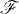# DISCRETE-TIME MODELS

In discrete-time models, it is assumed that trading takes place at discrete time points. In this chapter, we present discrete-time models of financial markets. In particular, we will introduce the binomial model.

# 41.1 Basic Concepts and Facts

Definition 41.1 (Discrete Market). Let T be a positive real number and N be a positive integer. Let 0 = t0 < t1 < ··· < tN = T. Let (Ω,, P) be a probability space, where Ω is finite,= 2Ω, and P{ω} > 0 for every ωΩ. A discrete market is a (d + 1)-dimensional stochastic processdefined on the probability space (Ω,, P).

The first entry S(0)n is the price at time tn of a riskless asset and is deterministic, that is,where rn > − 1 is the risk-free interest rate in the nth period [tn−1, tn].

For j ≥ 1, the jth entry S(j)n is the price at time tn of the jth risky asset and follows the following stochastic dynamics:

Get Measure, Probability, and Mathematical Finance: A Problem-Oriented Approach now with the O’Reilly learning platform.

O’Reilly members experience live online training, plus books, videos, and digital content from nearly 200 publishers.